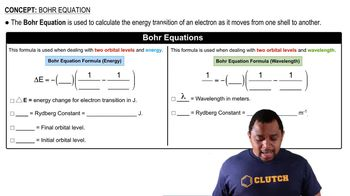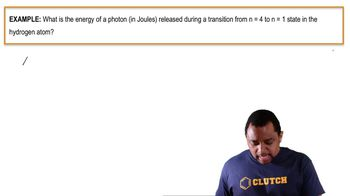Start typing, then use the up and down arrows to select an option from the list.
15:44 minutes
Problem 69
Textbook Question

# Calculate the wavelength of the light emitted when an electron in a hydrogen atom makes each transition and indicate the region of the electromagnetic spectrum (infrared, visible, ultraviolet, etc.) where the light is found. a. n = 2¡n = 1 b. n = 3¡n = 1 c. n = 4¡n = 2 d. n = 5¡n = 2Verified Solution
This video solution was recommended by our tutors as helpful for the problem above.
294views